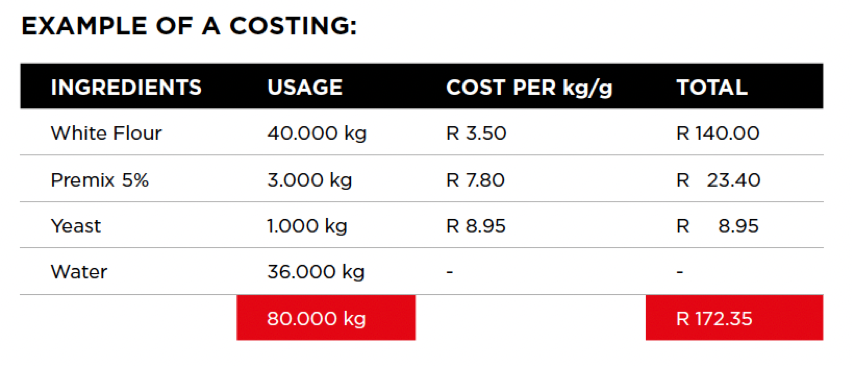### BakingGlossary

#### How To Cost A Recipe

In an attempt to simplify and demonstrate the benefits of effective costing, apply the steps below. You will be able to accurately forecast costs and potential profits allowing for efficient planning and management of your business or department.

Basic steps in calculating a cost price:

• List all the ingredients in the recipe, allocating a real cost price per ingredient.
• Work out the cost per kg on every ingredient.
• Write down the exact amount used (in grams) next to the ingredient used.
• Multiply the cost per kg by the amount required in the recipe. (This will equate to the total cost per ingredient).
• Add the total costs per ingredient to reach the total recipe/batch cost.
• Sum the total amount used for every ingredient to attain the total batch weight.
• Divide the scaling weight (e.g. 500 g) into the total batch weight to calculate the yield of the recipe.
• Divide the yield into the total batch cost to attain a cost per loaf of bread.Scaling weight: 500 g
Yield: (80.000/500) = 160
Cost per loaf: (172.35/160) = R 1.08
Selling per loaf: R 4.50
Gross profit: 76%